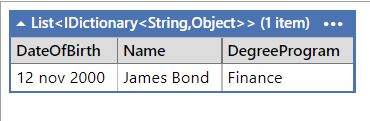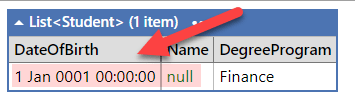Assume you have the following class:

``````public class Person
{
public DateTime DateOfBirth { get;}
public string Name { get; }
}
``````

Assume you also have the following class, that inherits from `Person`:

``````public class Student : Person
{
public string DegreeProgram { get; }
}
``````

There is an excellent library, Dapper, that you can use for mapping between C# objects and the database.

First, let us let Dapper create for us a dynamic object, and dump that using Linqpad.

To get a `dynamic` object when using the Query method, do not specify a type.

``````var cn = new SqlConnection("data source=;trusted_connection=true;database=test;encrypt=false");
var result = cn.Query("Select '12 nov 2000' DateOfBirth, 'James Bond' Name, 'Finance' DegreeProgram");
result.Dump();
``````

This will display the following:Next, we want to map the query to a strongly typed object.

Let us start with the `Person` class.

``````var cn = new SqlConnection("data source=;trusted_connection=true;database=test;encrypt=false");
var result = cn.Query<Person>("Select '12 nov 2000' DateOfBirth, 'James Bond' Name, 'Finance' DegreeProgram");
result.Dump();
``````

This will output the following:The problem arises when you try to map to a `Student` type.

``````var cn = new SqlConnection("data source=;trusted_connection=true;database=test;encrypt=false");
var result = cn.Query<Student>("Select '12 nov 2000' DateOfBirth, 'James Bond' Name, 'Finance' DegreeProgram");
result.Dump();
``````

This outputs the following:Notice that the `DateOfBirth` and `Name` are the default values of their respective types - DateTime.MinValue for a DateTime and `null` for a string.

If we modify the `Person` class and make the properties read/write:

``````public class Person
{
public DateTime DateOfBirth { get; set; }
public string Name { get; set; }
}
``````

If we re-run the query we get this:Now the data is being pulled correctly.

The problem here is that `Dapper` seems to be unable to set the properties of the parent after it has successfully done so for the child if the parent is immutable.

## Moral

When mapping objects with an inheritance chain in Dapper, beware if they are immutable.

The code is in my Github.

Happy hacking!